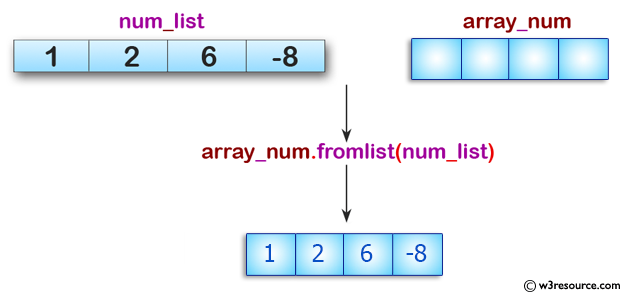﻿ Python: Append items from a specified list - w3resource# Python: Append items from a specified list

## Python: Array Exercise-9 with Solution

Write a Python program to append items from a specified list.

Pictorial Presentation:Sample Solution:

Python Code :

``````from array import *
num_list = [1, 2, 6, -8]
array_num = array('i', [])
print("Items in the list: " + str(num_list))
print("Append items from the list: ")
array_num.fromlist(num_list)
print("Items in the array: "+str(array_num))
``````

Sample Output:

```Items in the list: [1, 2, 6, -8]
Append items from the list:
Items in the array: array('i', [1, 2, 6, -8])
```

Python Code Editor:

What is the difficulty level of this exercise?

Test your Python skills with w3resource's quiz

﻿

## Python: Tips of the Day

Python: Unpack a Tuple

```>>> items = (0, 'b', 'one', 10,  11, 'zero')
>>> a, b, c, d, e, f = items
>>> print(f)
zero
>>> a, *b, c = items
>>> print(b)
['b', 'one', 10, 11]
>>> *_, a, b = items
>>> print(a)
11
```